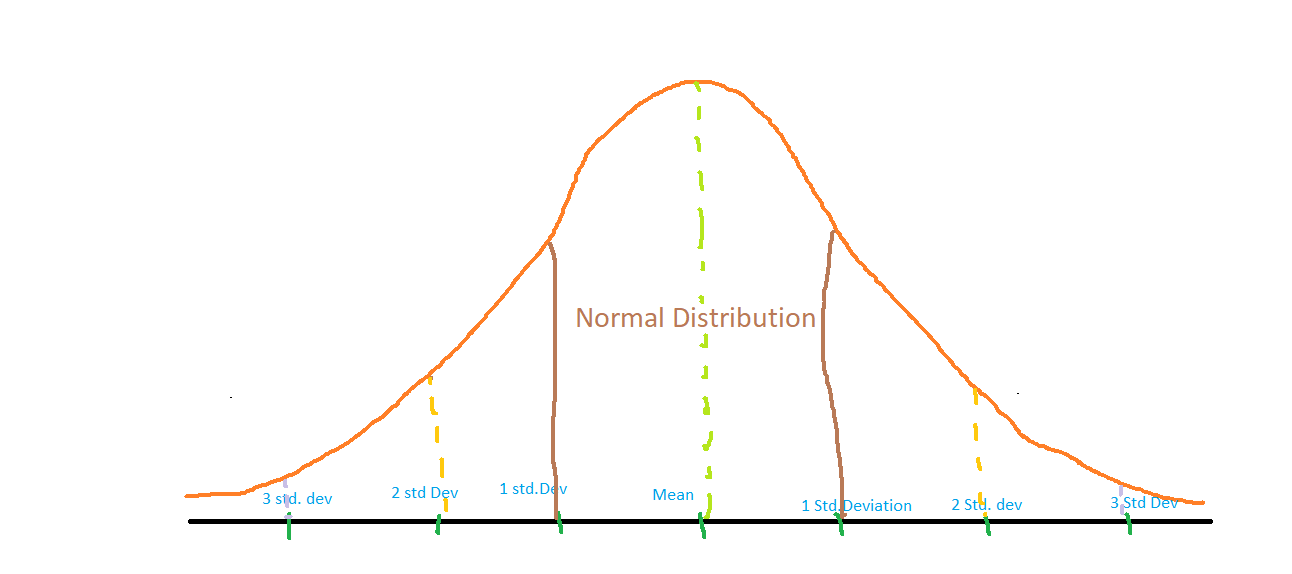# Z score for Outlier Detection – Python

• Difficulty Level : Medium
• Last Updated : 27 Aug, 2020

Z score is an important concept in statistics. Z score is also called standard score. This score helps to understand if a data value is greater or smaller than mean and how far away it is from the mean. More specifically, Z score tells how many standard deviations away a data point is from the mean.

Z score = (x -mean) / std. deviation

A normal distribution is shown below and it is estimated that
68% of the data points lie between +/- 1 standard deviation.
95% of the data points lie between +/- 2 standard deviation
99.7% of the data points lie between +/- 3 standard deviationZ score and Outliers:
If the z score of a data point is more than 3, it indicates that the data point is quite different from the other data points. Such a data point can be an outlier.
For example, in a survey, it was asked how many children a person had.
Suppose the data obtained from people is

1, 2, 2, 2, 3, 1, 1, 15, 2, 2, 2, 3, 1, 1, 2

Clearly, 15 is an outlier in this dataset.

Let us use calculate the Z score using Python to find this outlier.
Step 1: Import necessary libraries

 `import` `numpy as np `

Step 2: Calculate mean, standard deviation

 `data ``=` `[``1``, ``2``, ``2``, ``2``, ``3``, ``1``, ``1``, ``15``, ``2``, ``2``, ``2``, ``3``, ``1``, ``1``, ``2``]``mean ``=` `np.mean(data)``std ``=` `np.std(data)``print``(``'mean of the dataset is'``, mean)``print``(``'std. deviation is'``, std)`

Output:

```mean of the dataset is 2.6666666666666665
std. deviation is 3.3598941782277745```

Step 3: Calculate Z score. If Z score>3, print it as an outlier.

 `threshold ``=` `3``outlier ``=` `[]``for` `i ``in` `data:``    ``z ``=` `(i``-``mean)``/``std``    ``if` `z > threshold:``        ``outlier.append(i)``print``(``'outlier in dataset is'``, outlier)`

Output:

`outlier in dataset is `

Conclusion: Z score helps us identify outliers in the data.

My Personal Notes arrow_drop_up